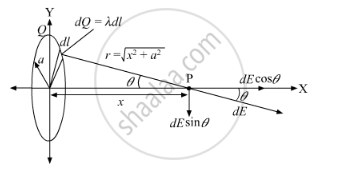# A Charge is Distributed Uniformly Over a Ring of Radius 'a'. Obtain an Expression for the Electric Intensity E at a Point on the Axis of the Ring. Hence, Show that for Points at Large Distance from the Ring, It Behaves like a Point Charge. - Physics

A charge is distributed uniformly over a ring of radius 'a'. Obtain an expression for the electric intensity E at a point on the axis of the ring. Hence, show that for points at large distance from the ring, it behaves like a point charge.

#### Solution

Here, we have a ring of radius a that carries a uniformly distributed positive total charge Q.We have to calculate the electric field due to a ring at a point P lying a distance x from its centre along the central axis perpendicular to the plane of the ring.

As the charge is distributed uniformly over the ring, the charge density over the ring can be written as follows:

lambda=Q/(2pia)

Along the x-axis, the perpendicular components of the electric fields due to charge on the ring cancel each other out.

As there is same charge on both sides of the ring, the magnitude of the electric field at P due to the segment of charge dQ is given by

dE=k_e (dQ)/r^2

E_x= int_"ring"k(dQ)/r^2costheta

=int_0^(2pia) k (lambdadl)/r^2 x/r

=klambda x/r^3 int_0^(2pia) dl

=klambda x/r^3 2pia

=kQ/(2pia)x/r^3 2pia

=KQx/r^3=KQ x/sqrt((x^2+a^2))^3

1. At the centre of the ring, the electric field is zero as x = 0.

2. When  x >> , a can be neglected in the denominator compared to x, and the loop looks like a point charge at large distances.

E=kQ x/sqrt((x^2+a^2)^3)

E=kQ/x^2, ("at "x" >> "a)

Concept: Electric Field Due to a Point Charge
Is there an error in this question or solution?
2015-2016 (March) Delhi Set 1
Share